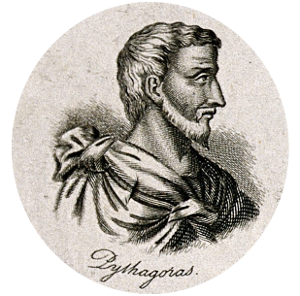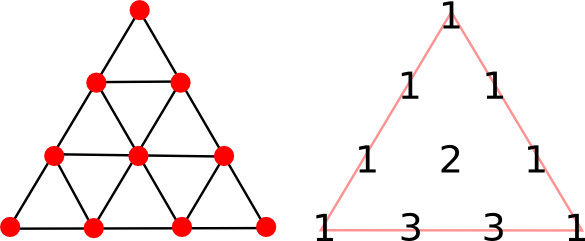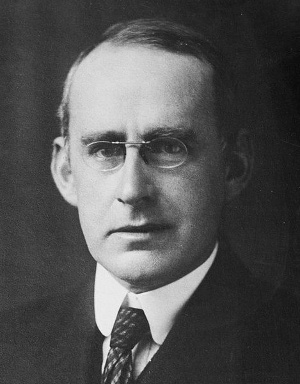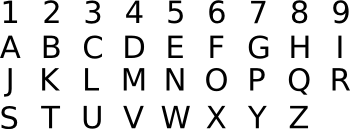### Numerology

March 2, 2015

The Greek philosopher, Pythagoras, is known to everyone for the Pythagorean theorem, but his greatest impact in his own time was as founder of the religious movement, Pythagoreanism. Little is known about this pre-Socratic philosopher, but it's thought that disciples of Pythagoreanism believed that numbers were the ultimate reality. This idea that reality is different from what we observe is a feature, also, of the philosophy of Plato, and it's conjectured that Plato was influenced by Pythagoras.Pythagoras (Πυθαγορας)
c. 570 - c. 495.

(From Mathematicians and astronomers: twenty portraits, engraving by J.W. Cook, 1825, via Wikimedia Commons.)

Pythagoras had the idea that 10 was the most perfect number, possibly through examination of his fingers and toes. Mathematicians have their own perfect numbers, which are numbers whose value equals that of all their divisors excluding themselves. Thus, ten is not a perfect number in today's sense, since 1 + 2 + 5 ≠ 10, but six is (1 + 2 + 3 = 6). Whether or not there are an infinity of perfect numbers is an unsolved problem in number theory.

The Pythagoreans represented the perfection of ten by a graphic, called the tetractys (τετρακτυς), as shown below.The tetractys (left) and the top of Pascal's triangle (right). (Illustration by the author, rendered using Inkscape.)

There was a lot of symbolism in the tetractys aside from the number of points adding to ten. The last row of four relates to the four classical elements, Earth, Air, Fire and Water.

As you can see from the figure, the tetractys acts as a template for the coefficients of the polynomial expansion of (x + 1)n; for example, the last row represents (x + 1)3 = 1x3 + 3x2 + 3x1 + 1x0. This triangular array of coefficients is known as Pascal's triangle, named after the French mathematician, Blaise Pascal. The coefficients are series A007318 in the On-Line Encyclopedia of Integer Sequences. I wrote about Pascal in a previous article (Blaise Pascal, June 19, 2012).

Physics is typically the realm of numbers with dimensions that are mixtures of powers of length, mass, time, and sometimes charge. That's why the predominant system of measurement before the International System of Units was "MKS," for meter-kilogram-second. However, it's possible to arrange fundamental physical constants to yield dimensionless numbers. One example of this is the fine structure constant, denoted by the Greek letter, α.

The fine structure constant is related to some rather fundamental things; namely, the elementary charge e, Planck's constant h, the speed of light c and the mathematical constant π,
α = (2 π e2)/(h c)
This constant, which is quite close to the
reciprocal of 137 (~1/137.036), expresses the strength of the interaction of charged particles. It's fundamental to electromagnetism, and it combines those three fundamental physical constants, e, h and c. At one time, its calculated value was very close to 1/136. Now, it's very close to 1/137.

Eminent
physicist, Arthur Eddington, thought that there was something "magical" about this number, and he proposed some a priori reasons why it should have that value. Eddington continued to investigate dimensionless numbers, deriving an a priori value for the number of protons in the universe. This number, now called the Eddington number, is calculated to be 136 x 2256, or 137 x 2256, or about 1080. Paul Dirac made similar observations.Physicist, Sir Arthur Stanley Eddington (1882-1944)

(United States Library of Congress, Prints and Photographs Division, image ggbain.38064, via Wikimedia Commons.)

To most physicist of the time, all this seemed to be a retreat, from the way modern physics is practiced, to the philosophy of Aristotle. Some compared it to the ancient practice of numerology. In fact, Guido Beck, Hans Bethe, and Wolfgang Riezler published a parody of this approach in the respected physics journal, Die Naturwissenschaften,[1-2] in which they related the dimensioned value of absolute zero temperature, T0 = -273 °C, to the fine structure constant; viz.,
T0 = -(2/α - 1)

Since many people still believe in "lucky numbers," (not to be confused with a mathematician's lucky numbers) it's interesting to see how words are transformed into single digits. You can associate the digits, 0-9, with letters of the alphabet, as shown in the table. All this is rather arbitrary, but we're not operating in the realm of science when we do numerology.We can then add together the numbers associated with every letter in a word. Taking Tikalon as an example, 2 + 9 + 2 + 1 + 3 + 6 + 5 = 28. If we're interested in just a single digit, we do repeated partitions and sums to yield 2 + 8 = 10, and 1 + 0 = 1. Thus, Tikalon's "lucky number" is one. As a scientist, I'm not inclined to play 111 in the New Jersey State Lottery; or, for that matter, play the lottery at all.

In a recent paper on arXiv, mathematicians Steve Butler, Ron Graham, and Richard Stong examine this "partition and sum" process. They treat a more general case in which just a single plus sign is inserted into the number, or as many as one plus sign between every digit, and they consider numbers written in binary (base-two) format. They are able to show that very few iterations of such operations are needed to get down to the single digit, one.

In examining the bound on how many steps it takes to get a binary number down to 1, they observe that inserting as many plus signs as possible (that is, summing all the digits) for a number n results in a new number of the order log(n). The problem then reduces to how long it takes for log(log(· · · (log(n)) · · · )) to get to a value less than one. As you can see, you might need an infinite number of iterations, but the number of iterations grows very much more slowly than n.

### References:

Linked Keywords: Ancient Greek philosophy; Greek philosopher; Pythagoras; Pythagorean theorem; religious movement; Pythagoreanism; pre-Socratic philosopher; natural number; reality; Plato; Wikimedia Commons; finger; toe; mathematician; perfect number; divisor; infinity; unsolved problem in number theory; tetractys; Pascal's triangle; Inkscape; symbol; symbolism; classical elements; Earth; Air; Fire; Water; template; coefficient; polynomial expansion; triangle; triangular; Pascal's triangle; French; Blaise Pascal; A007318; On-Line Encyclopedia of Integer Sequences; physics; dimension; exponentiation; power; length; mass; time; electric charge; International System of Units; MKS system of units; fundamental physical constant; dimensionless number; fine structure constant; Greek alphabet; Greek letter; elementary charge; Planck's constant; speed of light; multiplicative inverse; reciprocal; Coulomb's law; interaction of charged particles; electromagnetism; physicist; Arthur Eddington; a priori; proton; universe; Eddington number; Paul Dirac; Dirac large numbers hypothesis; Aristotle; numerology; Guido Beck; Hans Bethe; Wolfgang Riezler; parody; scientific journal; Die Naturwissenschaften; absolute zero temperature; lucky number; numerical digit; letters of the alphabet; science; Tikalon; New Jersey State Lottery; lottery; arXiv; Steve Butler; Ron Graham; Richard Stong; binary number; iteration; bounded set; bound; natural logarithm; log(n); infinity; infinite number; G. Beck, H. Bethe, and W. Riezler, "Remarks on the quantum theory of the absolute zero of temperature," Die Naturwissenschaften, vol. 2 (1931), p. 39.